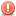1. ##Help!!Duplicate statements printed

I'm matching the value of each of the 2D array against 1D Array. 1D array is actually the conversion of 2D to 1D array, so that i can use Arrays.sort() to sort the array to ascending order. However when i'm printing out, it give me extra values. I should have 6 values printed out only.
Code:
``` In score 2D array:
[ i ] [ j ]    [ value ]
0    0          1.0
0    1          0.04
1    0          0.24
1    1          1.0
2    0          0.15
2    1          0.14```
Code:
```Before sorting newArray 1D array:
[ a ]          [ value ]
0               1.0
1               0.24
2               0.24
3               1.0
4               0.15
5              0.14```
Code:
```After sorting newArray 1D array:
[ a ]           [ value ]
0               0.14
1               0.15
2               0.24
3               0.24
4               1.0
5               1.0```
Code:
```The output is:
[ i ] [ j ]      newArray[a]
2    1            0.14
2    0            0.15
0    1            0.24
1    0            0.24
0    1            0.24  //this is repeated
1    0            0.24  //this is repeated
0    0             1.0
1    1             1.0
0    0             1.0   //this is repeated
1    1             1.0  //this is repeated```
Here is my code:
Code:
```queryIDArray(length of 3) and srnIDArray(length of 2) are all 1D arrays.
public static void sortArray(float[][] score)
{
int k=0;

float[] newArray = new float[queryIDArray.length*scrnIDArray.length];

for(int i=0;i<queryIDArray.length;i++)
{
for(int j=0;j<scrnIDArray.length;j++)
{
newArray[k] = score[i][j];
k++;
}
}

Arrays.sort(newArray);
for(int a=0;a<newArray.length;a++)
{
for(int i=0;i<queryIDArray.length;i++)
{
for(int j=0;j<scrnIDArray.length;j++)
{

if(newArray[a]==score[i][j])
System.out.println(i+" "+j+" "+newArray[a]);
//what condition should I put so that it will not repeat?

}
}
}
}```
How can I check if the value is printed out once, it would not print it out again?Reply With Quote

2. ## Re: Help!!Duplicate statements printed

I don't get what you're trying to do, but it looks like the completely wrong approach to me anyway.Reply With Quote

3. ##Re: Help!!Duplicate statements printed

Hmm, for example, if i have an 2D array that is float[x][y] score = {1.0, 0.3, 1.0, 0.2}. 'x' and 'y' are positions of the floating value. In this case, score of 1.0 is at score, scoreof 0.3 is at score and so on. Now, I put the score array into 1D array so that i can use Arrays.sort() to sort the 2D array. Here is the code of conversion of 2D to 1D array.
Code:
```int k=0;
for(int x=0;x<1;x++)
{
for(int y=0;y<4;y++)
{
newArray[k] = score[x][y];
k++;
}
}```
Next, I sort the array using Arrays.sort(newArray). Now, i want to match the 1D array (i.e. newArray) with the 2D array (i.e. score) to get back the original positions (i.e. 'x' and 'y'). However, i have repeated values printed out as there are 2 "1.0" in both 1D and 2D arrays. Here is my code:
Code:
```for(int a=0;a<newArray.length;a++)
{
for(int x=0;x<4;x++)
{
for(int y=0;y<4;y++)
{
if(newArray[a]==score[x][y]) //matching 1D value to 2D value
System.out.println(x+" "+y+" "+newArray[a]);	//get positions

}
}
}```
This is my output:
Code:
```
[ x ]            [ y ]            sorted score
0                3                  0.2
0                1                  0.3
0                0                  1.0
0                2                  1.0 //should stop at here
0                0                  1.0  //redundant
0                2                  1.0   //redundant```
What extra code should I put so that when it had matched the 2 positions of "1.0" and then stop printing?Reply With Quote

4. ## Re: Help!!Duplicate statements printed

Since scores can be dupes, there is no hope that this can ever work.

What you need to do is write a small class containing three members: int x, y and float score. Let's call it Entry. You need to implement the Comparable interface for it and override Object.equals(). The implementations of compare() and equals() only act on the score member.
Then you create a linear array of Entry objects, giving each one the information from one entry of the 2d array.
Then you call sort() on the linear array. You're done.Reply With Quote

5. ##Re: Help!!Duplicate statements printed

Hmm, the suggestion you gave is abit too dfficult to do. Is there a way to sort 2D arrays straightaway using java predefined classes?Reply With Quote

6. ## Re: Help!!Duplicate statements printed

As you are matching on the basis of values.. and values are found twice in both arrays.. those will be printed twice...

so here i would suggest some modifications.. with the hope that those would work...

Code:
```Arrays.sort(values);
Vector v = new Vector();
for(int t=0;t<values.length;t++)
{
if(!v.contains(values[t]))
{
}
}

for(int a=0;a<v.size();a++)
{
for(int i=0;i<queryIDArray.length;i++)
{
for(int j=0;j<scrnIDArray.length;j++)
{

if(v[a]==score[i][j])
System.out.println(i+" "+j+" "+newArray[a]);
//what condition should I put so that it will not repeat?

}
}
}```Reply With Quote

7. ## Re: Help!!Duplicate statements printedOriginally Posted by huiling25
Is there a way to sort 2D arrays straightaway using java predefined classes?
No. Besides, no sorting mechanism available in Java preserves indices, which is what you want to do.

Hmm, the suggestion you gave is abit too dfficult to do.
Have you even tried it?Reply With Quote

####Posting Permissions

• You may not post new threads
• You may not post replies
• You may not post attachments
• You may not edit your posts
•

Featured